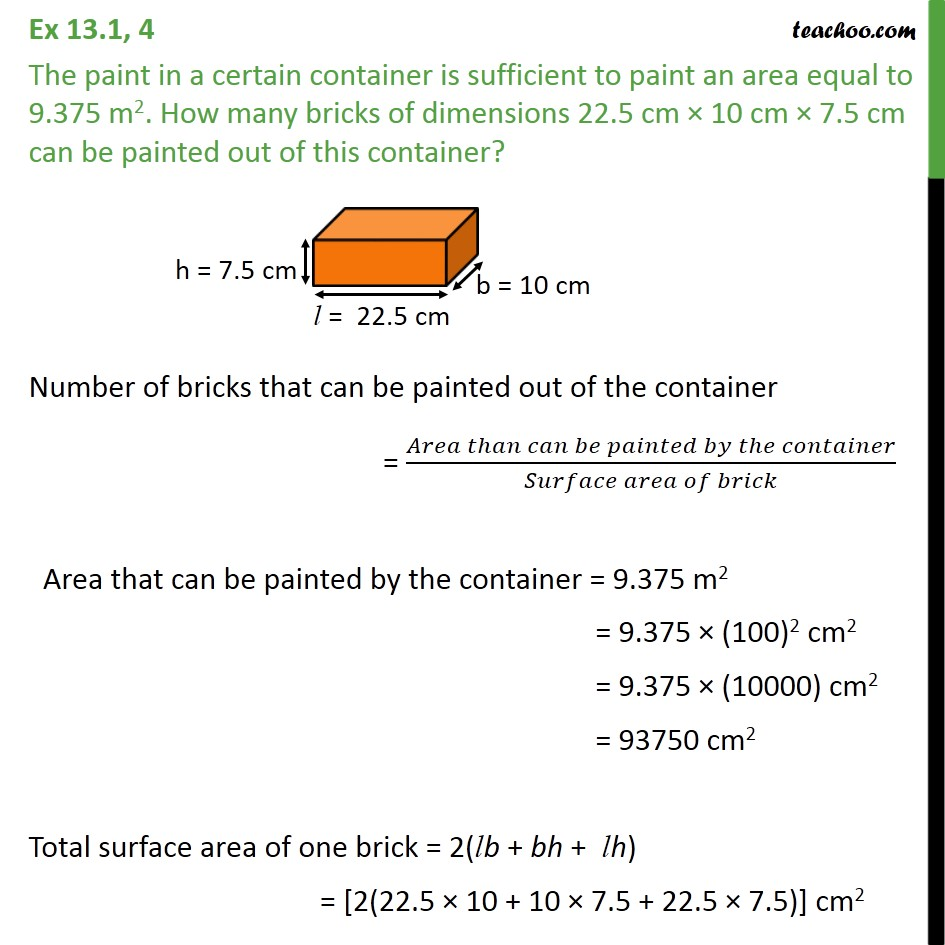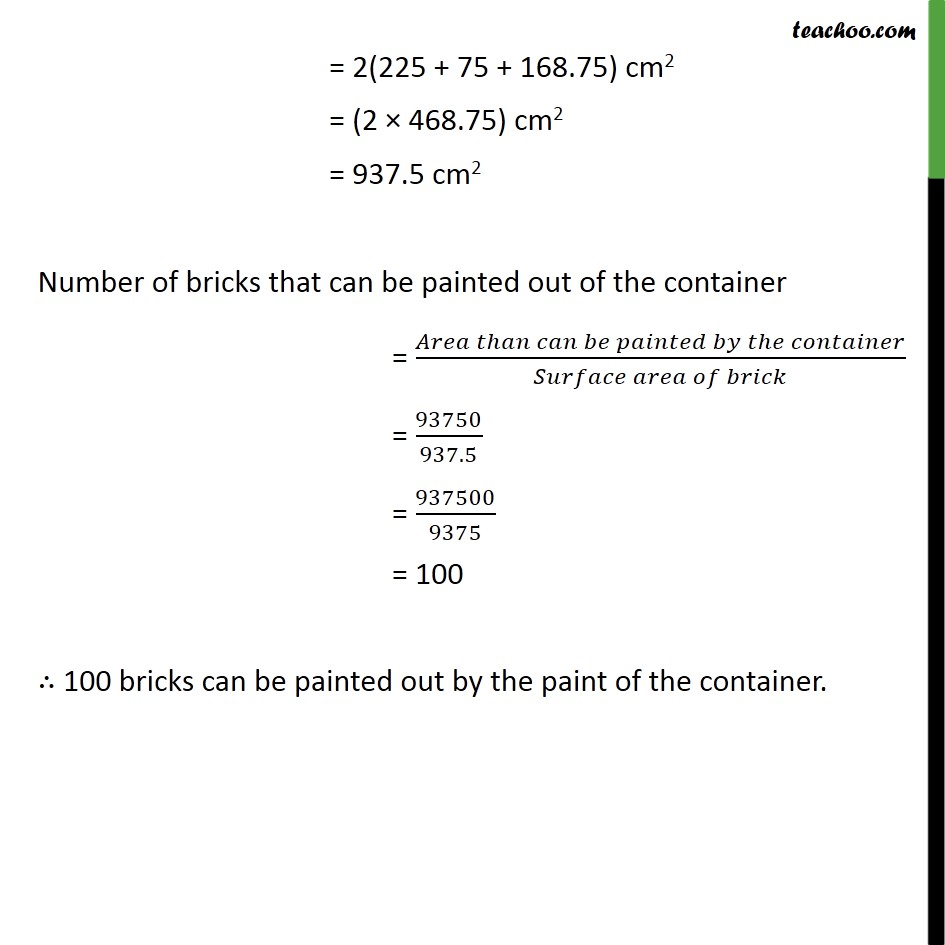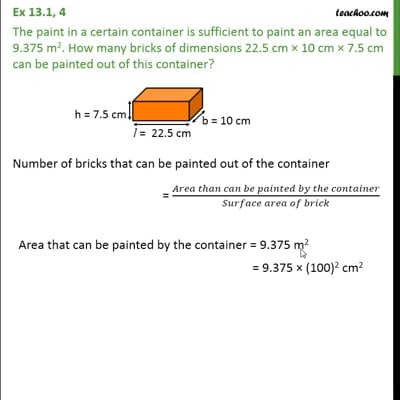Area Of Cube/ Cuboid

Chapter 13 Class 9 Surface Areas and Volumes
Concept wiseThis video is only available for Teachoo black users

Introducing your new favourite teacher - Teachoo Black, at only ₹83 per month

### Transcript

Ex 13.1, 4 The paint in a certain container is sufficient to paint an area equal to 9.375 m2. How many bricks of dimensions 22.5 cm 10 cm 7.5 cm can be painted out of this container? Number of bricks that can be painted out of the container = ( )/( ) Area that can be painted by the container = 9.375 m2 = 9.375 (100)2 cm2 = 9.375 (10000) cm2 = 93750 cm2 Total surface area of one brick = 2(lb + bh + lh) = [2(22.5 10 + 10 7.5 + 22.5 7.5)] cm2 = 2(225 + 75 + 168.75) cm2 = (2 468.75) cm2 = 937.5 cm2 Number of bricks that can be painted out of the container = ( )/( ) = 93750/937.5 = 937500/9375 = 100 100 bricks can be painted out by the paint of the container.